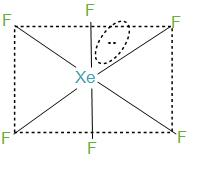QuestionAnswers

# The structure of $XeF{}_{6}$is: (A) pentagonal bipyramidal(B) distorted octahedral(C) capped octahedral(D) square pyramidalVerified
128.7k+ views
Hint: $XeF{}_{6}$ is a noble gas compound , which is stable at normal temperature. $XeF{}_{6}$ is having hybridisation that is $s{{p}^{3}}{{d}^{3}}$. It is found to have a lone pair of electrons.

- Firstly, we have to learn how to determine the type of hybridisation and understand the formation of a certain molecular geometry in xenon hexafluoride along with the bond angles.- We can determine the hybridisation in two ways:
Firstly, by knowing the number of bond pairs and lone pairs. During the formation of $XeF{}_{6}$, xenon has 8 electrons in its valence shell and it forms six bonds with the fluorine atoms and will have remaining two electrons. Further, we can say that the molecules will have one lone pair and 6 bond pairs. Now, if we take the steric number then it will be 7. And this can be interpreted as $s{{p}^{3}}{{d}^{3}}$.
- In the structure what happens here is that the fluorine atoms are placed in the vertices of the octahedron while lone pairs move in the space to avoid or we can say to reduce the repulsion. In simple words we can say that the six bond pairs are formed with fluorine and initially form a structure like octahedron but the lone pair that is present, gets its place in between the two fluorine atoms to reduce the repulsion. And hence, distort the structure of octahedrons, and therefore this structure is called a distorted octahedron.
-Secondly, we can also determine the hybridisation of xenon hexafluoride by using the common formula which is: $Hybridisation=\dfrac{1}{2}\times \left[ v+m-c+a \right]$
Here,
v =number of valence electrons,
m =monovalent
c =positive charge
a =negative charge
Let’s put the values according to the formula,
Hybridisation=
$Hybridisation=\dfrac{1}{2}\times \left[ 8+6-0+0 \right]$
\begin{align} & =\dfrac{1}{2}\times \left[ 14 \right] \\ & =7 \\ \end{align}
So we can say that, the hybridisation number is equal to 7. Now we can say that the hybridisation is $s{{p}^{3}}{{d}^{3}}$.
-VSEPR theory suggests the Pentagonal bipyramid geometry, but by electron diffraction experiments it is said to have octahedral geometry with fluorine atoms on the corners.
- Hence we can say that the option (a) and (b) both are correct.

- It is found that the complete hydrolysis of $XeF{}_{6}$produces$Xe{{O}_{3}}$ which is xenon trioxide. This trioxide is highly explosive and acts as a powerful oxidising agent in solution.
-It is also seen that $XeF{}_{6}$ is the strongest fluorinating agent.
- It is to be noted that $XeF{}_{6}$ is having hybridisation that is $s{{p}^{3}}{{d}^{3}}$. During the formation of, $XeF{}_{6}$ xenon has 8 electrons in its valence shell and it forms six bonds with the fluorine atoms and will have remaining two electrons. This is having distorted octahedral geometry.